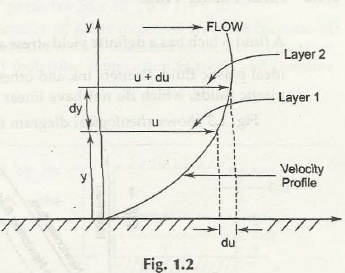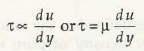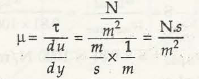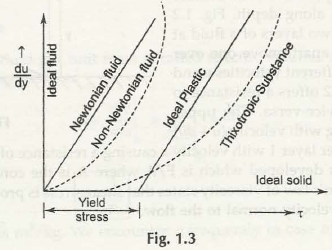Fluid can be classified into different categories. Some of the common classification of fluids includes:

1. Ideal Fluid
2. Real Fluid
3. Newtonian Fluid
4. Non-Newtonian Fluid
5. Ideal Plastic Fluid

Ideal Fluid:

Ideal fluids are the imaginary fluid with no viscosity ( = 0) and are also incompressible ( = 0). There is no such fluid which is having zero viscosity as all existing fluids are viscous in nature.

Real Fluid:

Real fluids are the existing fluid with viscosity. All existing fluids are viscous in nature and are termed as real fluids.

Newtonian Fluid:

The fluid which obeys Newton’s law of viscosity is known as Newtonian fluid.

According to Newton’s law of viscosity, “Shear stress of all real fluids is directly proportional to the velocity gradient or rate of shear strain.”

Let us consider viscous flow of a fluid over a solid surface with velocities u and (u + du) at two different layers Layer 1 and Layer 2 respectively. The resistance at the solid surface will be zero and it will increase as the depth increases as shown in Fig. 1.2Suppose the fluid is flowing in the direction of positive x and whose velocity profile gives a curve shape.

du shows the change in velocity as per Layer 1 and Layer 2

dy be the distance between these two layers 1 and 2

Mathematically,

Shear stress between these two layers can be written as-Where,

τ = Shear Stress

μ = Co-efficient of Viscosity

Note:

S.I. unit of co-efficient of viscosity is Ns/m2

C.G.S unit of viscosity is poise

1 poise = 0.1 Ns/m2

For example:

Water and air are the Newtonian fluids as both these possess some viscosity.

water = 10-3 Ns/m2

air = 1.81 x 10-5 Ns/m2

Non-Newtonian Fluid:

The fluid which does not obey Newton’s law of viscosity is known as Non-Newtonian fluid. In other words, the fluids in which, shear stress is not proportional to velocity gradient or rate of shear strain is known as Non-Newtonian Fluids.

Ideal Plastic Fluid

Fluid which has a linear relation of shear stress and velocity gradient along with higher yield value as compare to shear stress is known as ideal plastic fluid.

Classification of fluids is shown in the Fig. 1.3:This graph is plotted keeping the shear stress on x-axis and velocity gradient on y-axis.

Links of Next Mechanical Engineering Topics:-### Customer Reviews

My Homework Help
Rated 5.0 out of 5 based on 510 customer reviews at